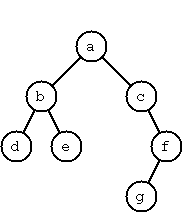Logic Programming WhatversusHow

 Assignment - Trees

 A binary tree is either empty or it is composed of a root element and two successors, which are binary trees themselves. See the example below.We can represent the empty tree by the atom nil and a non-empty tree by the term t(L,X,R), where X denotes the value of the node and L and R denote its left and right subtrees, respectively. The tree above would be written as shown below. t(t(t(nil,d,nil),b,t(nil,e,nil)),a,t(nil,c,t(t(nil,g,nil),f,nil))) A binary search tree is one in which every entry in the left branch is less than the value of the root node and the root node is less than every entry in the right branch. (In a balanced binary search tree, the are additional constraints in the heights or the numbers of nodes in the two branches of a binary search tree. However, in this assignment, we ignore these issues. The only thing that matters is the ordering property just mentioned.) Your first job on this assignment is to define a ternary predicate add in which the first argument is an arbitrary number, in which the second argument is a binary search tree, and in which the last argument is the result of adding the specified number to the specified binary search tree. For example, using your definition, when asked to compute add(2,t(nil,1,nil),T), Sierra should produce add(2,t(nil,1,nil),t(nil,1,t(nil,2,nil))). You second job is to use add to define a binary predicate binarize, in which the first argument is a list of natural numbers and the second argument is a representation of the numbers in the list as a binary search tree. For example, your program should convert the list [3,2,5,7,1] into the tree shown below. t(t(t(nil, 1, nil), 2, nil), 3, t(nil, 5, t(nil, 7, nil)))